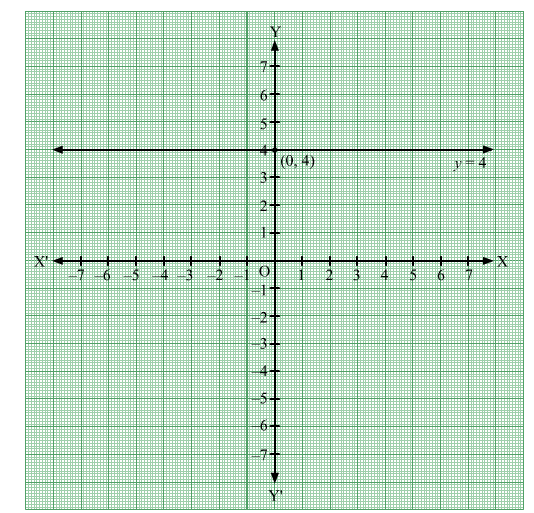# Draw the graph of each of the following equations.

Question:

Draw the graph of each of the following equations.
(i) = 4
(ii) + 4 = 0
(iii) y = 3
(iv) y = –3
(v) = –2
(vi) = 5
(vii) + 5 = 0
(viii) y = 4

Solution:

(i) The equation of given line is x = 4. This equation does not contain the term of y. So, the graph of this line is parallel to y-axis passing through the point (4, 0).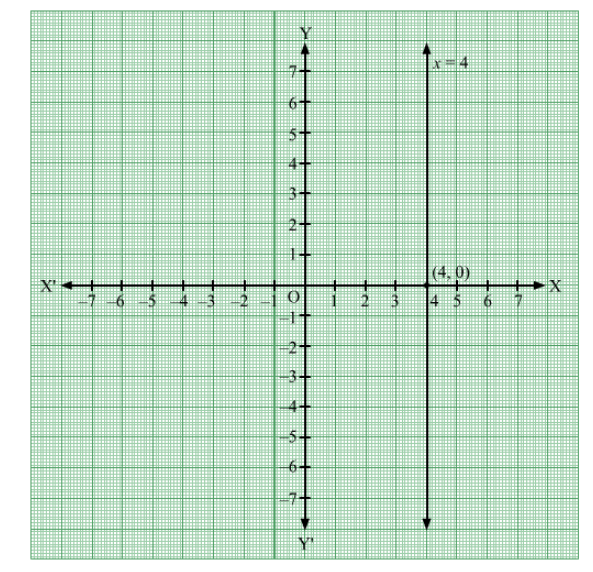(ii) The equation of given line is x + 4 = 0 or x = –4. This equation does not contain the term of y. So, the graph of this line is parallel to y-axis passing through the point (–4, 0).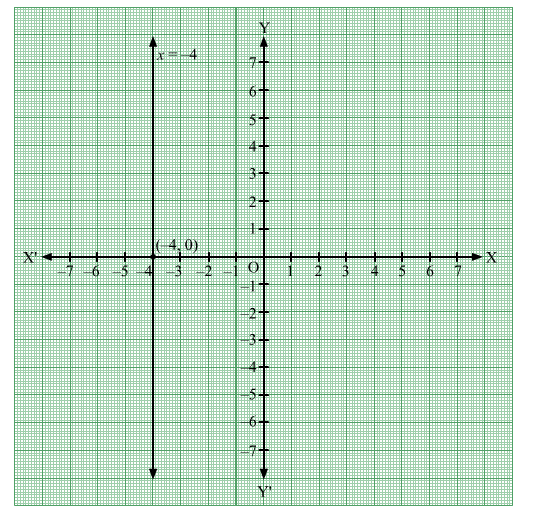(iii) The equation of given line is y = 3. This equation does not contain the term of x. So, the graph of this line is parallel to x-axis passing through the point (0, 3).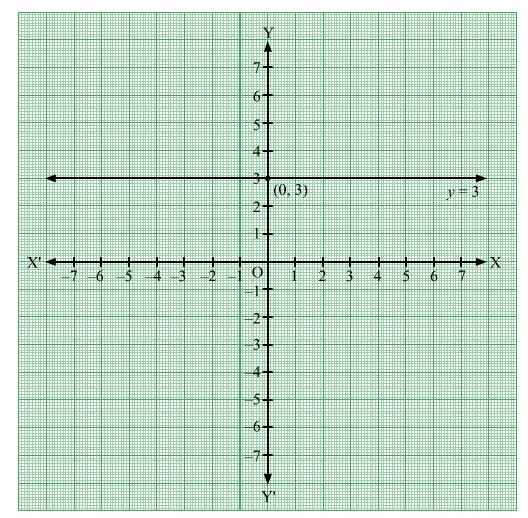(iv) The equation of given line is y = –3. This equation does not contain the term of x. So, the graph of this line is parallel to x-axis passing through the point (0, –3).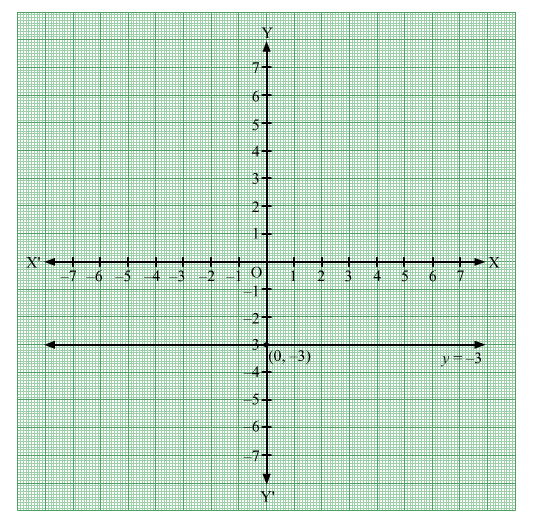(v) The equation of given line is x = –2. This equation does not contain the term of y. So, the graph of this line is parallel to y-axis passing through the point (–2, 0).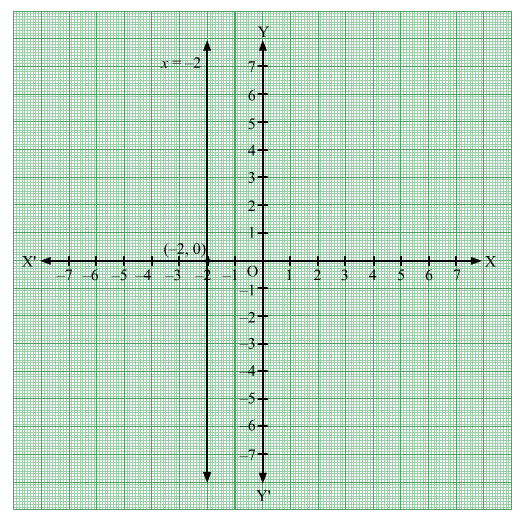(vi) The equation of given line is x = 5. This equation does not contain the term of y. So, the graph of this line is parallel to y-axis passing through the point (5, 0).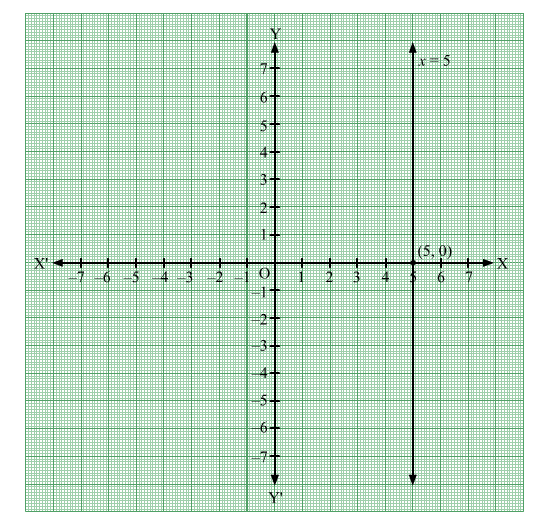(vii) The equation of given line is y + 5 = 0 or y = –5. This equation does not contain the term of x. So, the graph of this line is parallel to x-axis passing through the point (0, –5).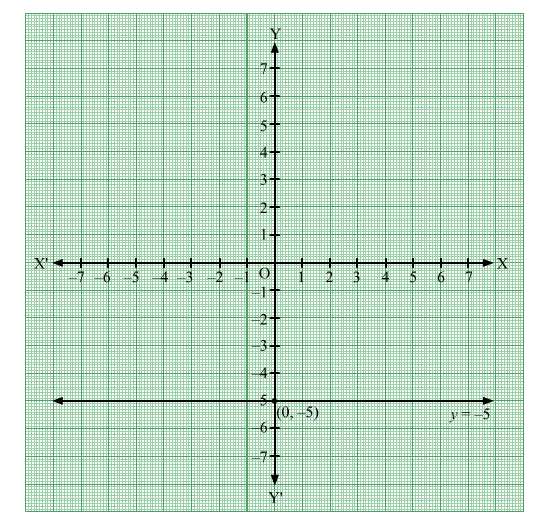(viii) The equation of given line is y = 4. This equation does not contain the term of x. So, the graph of this line is parallel to x-axis passing through the point (0, 4).